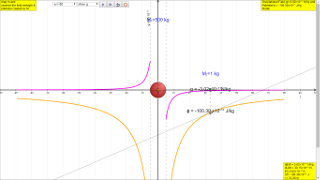gravity07

7.3.8 Relationship between F and U; between g and ϕ

To understand how g is related to ϕ:

1. Similarly, compare $g=-\genfrac{}{}{0.1ex}{}{GM}{{r}^{2}}$ and $\text{ϕ}=-\frac{\text{GM}}{r}$ in the above table.
2. If we differentiate $\text{ϕ}=-\frac{\text{GM}}{r}$    with respect to r, we will get  $\genfrac{}{}{0.1ex}{}{d\varphi }{dr}=-\genfrac{}{}{0.1ex}{}{GM}{\left(-{r}^{2}\right)}$, which has the same expression as g.
3. Hence, mathematically $\genfrac{}{}{0.1ex}{}{d\varphi }{dr}=\genfrac{}{}{0.1ex}{}{GM}{{r}^{2}}=-g$
4. To understand the meaning of $g=-\genfrac{}{}{0.1ex}{}{d\varphi }{dr}$ observe the two graphs carefully, on the right side where r is positive, the gradient of ϕ vs r graph is positive but the value of g will be negative. And on the left side where r is negative, the gradient of ϕ vs r graph is negative but the value of g is positive. Thus, $g=-\genfrac{}{}{0.1ex}{}{d\varphi }{dr}$

Similarly, it can be concluded that by multiplying both sides by test mass m.

$mg=-\genfrac{}{}{0.1ex}{}{dm\varphi }{dr}$
thus $F=-\genfrac{}{}{0.1ex}{}{dm\varphi }{dr}$

7.3.8.1 Activity To do

ICT inquiry worksheet 1 (E), as well as the "G field and potential" EJS here. The HTML5 version is below, the Java worksheet customization to HTML5 is work in progress.

7.3.8.2 Summary

 symbol $g=-\genfrac{}{}{0.1ex}{}{GM}{{r}^{2}}$ $\text{ϕ}=-\frac{\text{GM}}{r}$ name Field strength Potential units N kg-1 or m s-2 J kg-1 meaning Gravitational force per unit mass Gravitational potential energy per unit mass quantity vector scalar equation $\mathrm{|g|}=\genfrac{}{}{0.1ex}{}{GM}{{r}^{2}}$ towards the centre of the source mass $\text{ϕ}=-\frac{\text{GM}}{r}$ relationship to mass Force, $F=\frac{G{M}_{1}{M}_{2}}{{r}^{2}}$ = mg Potential energy, $U=-m\genfrac{}{}{0.1ex}{}{GM}{r}$ = mϕ graph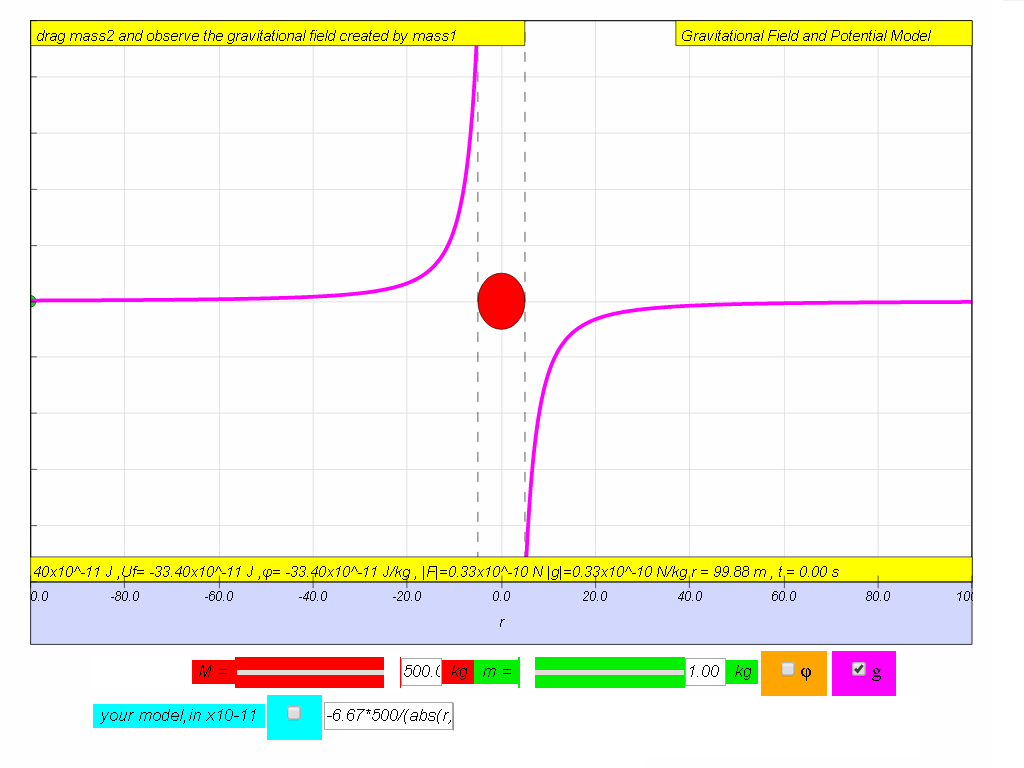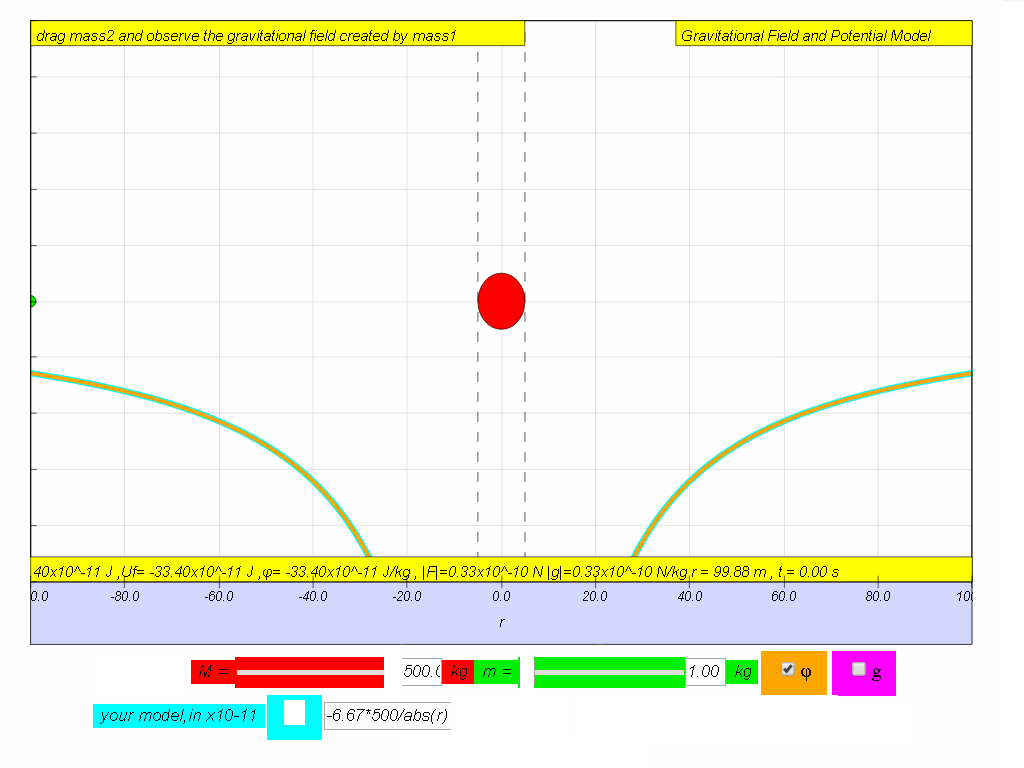computer model if M = 500. -6.67*500/(abs(r)*r) -6.67*500/abs(r) relationship between g and ϕ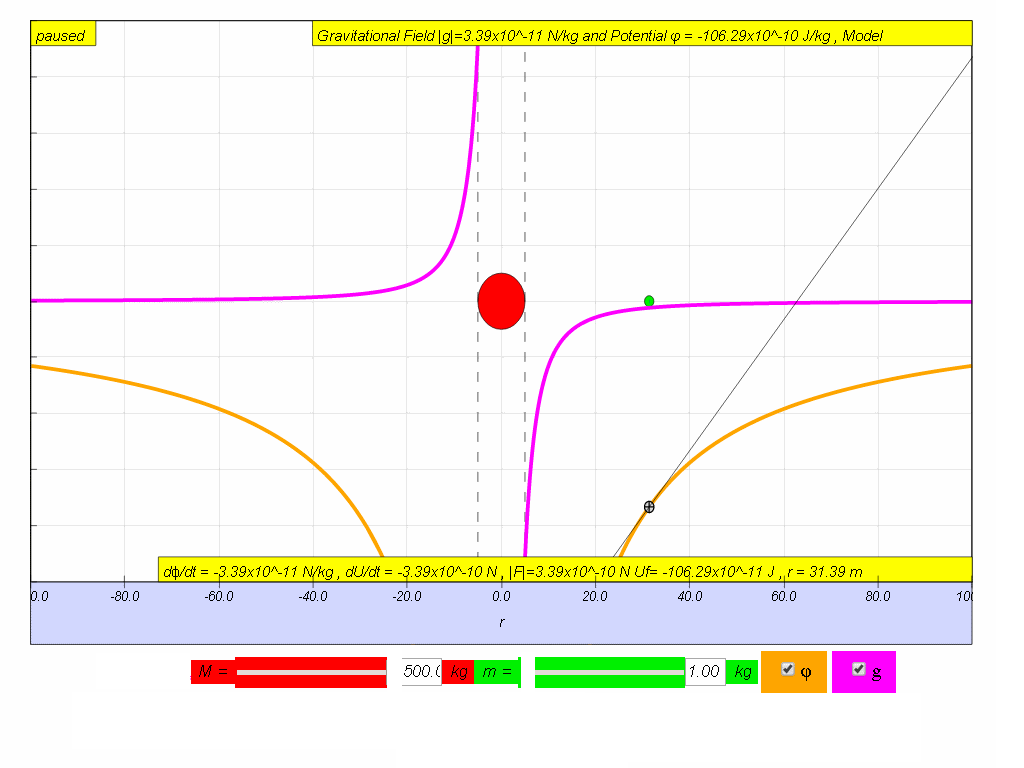$g=-\genfrac{}{}{0.1ex}{}{d\varphi }{dr}$ relationship between F and U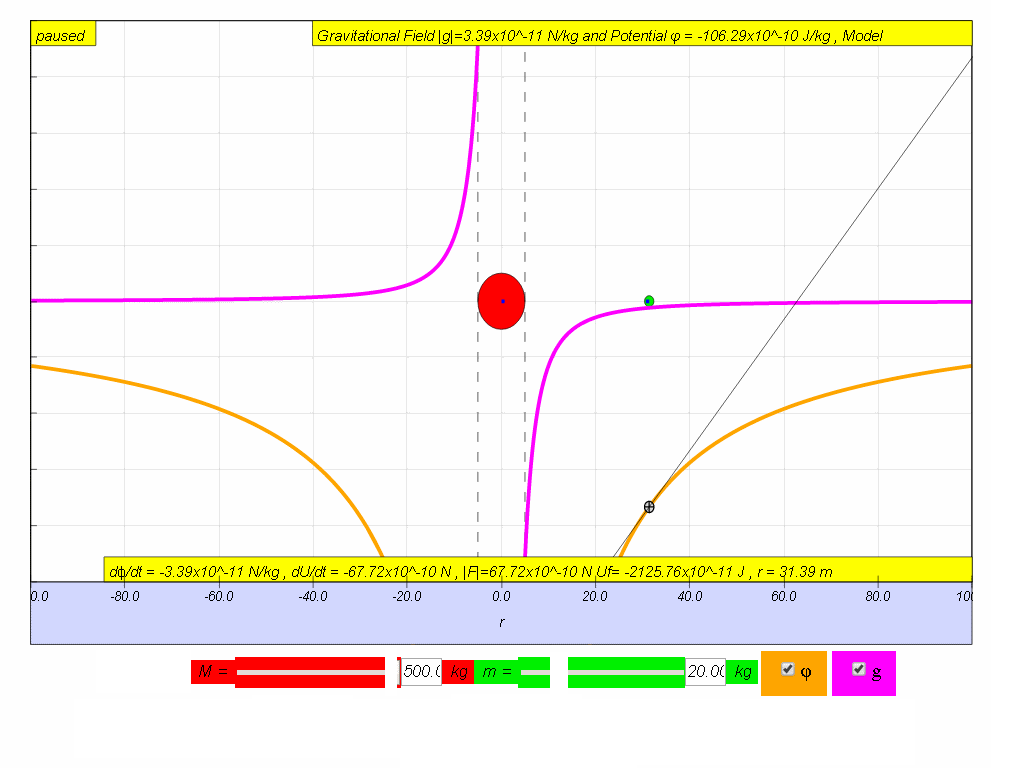$F=-\genfrac{}{}{0.1ex}{}{dU}{dr}$

Translations

Code Language Translator RunSoftware Requirements

SoftwareRequirements

 Android iOS Windows MacOS with best with Chrome Chrome Chrome Chrome support full-screen? Yes. Chrome/Opera No. Firefox/ Samsung Internet Not yet Yes Yes cannot work on some mobile browser that don't understand JavaScript such as..... cannot work on Internet Explorer 9 and below

Credits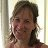This email address is being protected from spambots. You need JavaScript enabled to view it.; Anne Cox; Wolfgang Christian; Francisco Esquembre

end faq

http://iwant2study.org/lookangejss/02_newtonianmechanics_7gravity/ejss_model_gravity07/gravity07_Simulation.xhtml

Testimonials (0)

There are no testimonials available for viewing. Login to deploy the article and be the first to submit your review!